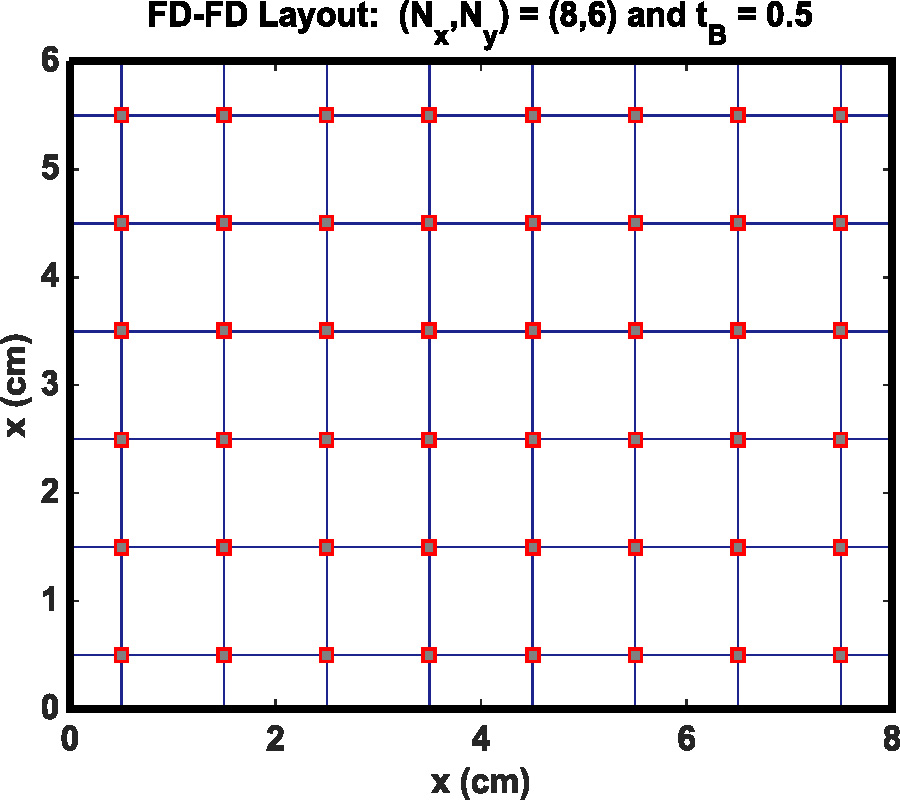# Vol. 92

Front:[PDF file] Back:[PDF file]
Latest Volume
All Volumes
All Issues
2019-04-27

#### Flexible Compact High-Order FD-FD Algorithm for Computing Mode Fields of Microwave Waveguides with Regular and Reentrant Corners

By Sin-Yuan Mu and Hung-Wen Chang
Progress In Electromagnetics Research C, Vol. 92, 151-164, 2019
doi:10.2528/PIERC19021802

## Abstract

We present a highly accurate frequency-domain finite-difference algorithm for computing mode field solutions of microwave waveguides with regular and reentrant corners. Based on FBS (Fourier-Bessel series)-derived 3-by-3 compact coefficients, our method allows for a flexible layout of the 2-D uniform grids so that distance from the waveguide boundaries to the adjacent unknowns can be arbitrary. Fourth to sixth-order convergent rates of the proposed coefficients are verified by resonance-frequency error analysis for rectangular microwave waveguides for both TE/TM polarizations. We also study the first four Neumann/Dirichlet eigenvalues of the L-shaped MW-WGs calculated by the flexible scheme, and the Neumann results are reported for the first time. Although our results achieve sixth-order accuracy for analytic modes, the order of accuracy is about one and a third for both fundamental TE and TM modes due to singularity around the reentrant corner.

## Citation

Sin-Yuan Mu and Hung-Wen Chang, "Flexible Compact High-Order FD-FD Algorithm for Computing Mode Fields of Microwave Waveguides with Regular and Reentrant Corners," Progress In Electromagnetics Research C, Vol. 92, 151-164, 2019.
doi:10.2528/PIERC19021802
http://jpier.org/PIERC/pier.php?paper=19021802

## References

1. Motz, H., "The treatment of singularities of partial differential equations by relaxation methods," Quart. Appl. Math., Vol. 4, 371-377, 1947.
doi:10.1090/qam/18442

2. Arfken, G. B. and H. J. Weber, Mathematical Methods for Physicists, Elsevier Academic Press, 2005.

3. Antipov, Y. A. and V. V. Sivestrov, "Diffraction of a plane wave by a right-angled penetrable wedge," Radio Science, Vol. 42, RS4006, 2007.
doi:10.1029/2007RS003646

4. Hadley, G. R., "High-accuracy finite difference equations for dielectric waveguide analysis I: Uniform regions and dielectric interfaces," Journal of Lightwave Technology, Vol. 20, No. 7, 1210-1218, 2002.
doi:10.1109/JLT.2002.800361

5. Chang, H.-W. and S.-Y. Mu, "Semi-analytical solutions of the 2-D homogeneous Helmholtz equation by the method of connected local fields," Progress In Electromagnetics Research, Vol. 109, 399-424, 2010.
doi:10.2528/PIER10092807

6. Hadley, G. R., "High-accuracy finite difference equations for dielectric waveguide analysis II: Dielectric corners," Journal of Lightwave Technology, Vol. 20, No. 7, 1219-1231, 2002.
doi:10.1109/JLT.2002.800371

7. Magura, S., S. Petropavlovsky, S. Tsynkov, and E. Turkel, "High-order numerical solution of the Helmholtz equation for domains with reentrant corners," Applied Numerical Mathematics, Vol. 118, 87-116, 2017.
doi:10.1016/j.apnum.2017.02.013

8. Pozar, D. M., Microwave Engineering, John Wiley & Sons, Inc., 2012.

9. Fox, L. and R. Sankar, "Boundary singularities in linear elliptic differential equations," J. Inst. Math. Applic., Vol. 5, 340-350, 1969.
doi:10.1093/imamat/5.3.340

10. Singer, I. and E. Turkel, "High-order finite difference methods for the Helmholtz equation," Comput. Methods Appl. Mech. Engrg., Vol. 163, 343-358, 1998.
doi:10.1016/S0045-7825(98)00023-1

11. Nabavi, M., "A new 9-point sixth-order accurate compact finite difference method for the Helmholtz equation," Journal of Sound and Vibration, Vol. 307, 972-982, 2007.
doi:10.1016/j.jsv.2007.06.070

12. Mu, S.-Y. and H.-W. Chang, "Theoretical foundation for the method of connected local fields," Progress In Electromagnetics Research, Vol. 114, 67-88, 2011.

13. Mu, S.-Y. and H.-W. Chang, "Dispersion and Local-Error Analysis of Compact LFE-27 Formula for Obtaining Sixth-Order Accurate Numerical Solutions of 3D Helmholtz Equation," Progress In Electromagnetics Research, Vol. 143, 285-314, 2013.
doi:10.2528/PIER13090103

14. Yuan, Q. and Z. He, "Bounds to eigenvalues of the Laplacian on L-shaped domain by variational methods," Journal of Computational and Applied Mathematics, Vol. 233, 1083-1090, 2009.
doi:10.1016/j.cam.2009.08.114

15. Jones, R. S., "Computing ultra-precise eigenvalues of the Laplacian within polygons," Advances in Computational Mathematics, Vol. 43, 1325-1354, 2017.
doi:10.1007/s10444-017-9527-y

16. Fox, L., P. Henrici, and C. Moler, "Approximations and bounds for eigenvalues of elliptic operators," SIAM J. NUMER. ANAL., Vol. 4, No. 1, 89-102, 1967.
doi:10.1137/0704008

17. Liu, X.-F. and S. Oishi, "Verified eigenvalue evaluation for the Laplacian over polygonal domains of arbitrary shape," SIAM J. Numer. Anal., Vol. 51, No. 3, 1634-1654, 2013.
doi:10.1137/120878446